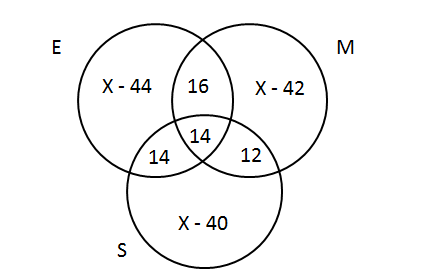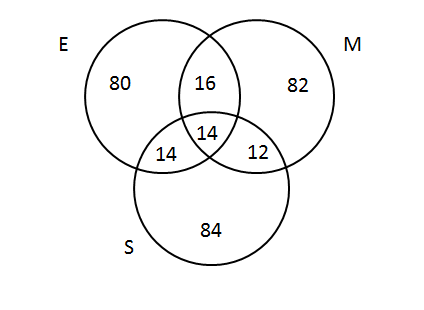# CAT Quantitative Aptitude Questions | CAT Set theory Questions - Union and Intersection

###### CAT Questions | Set theory | Union and IntersectionCAT Questions

The question is from CAT Set theory. It is about venn diagrams. We just need to draw the diagram without any mistakes. CAT exam is known to test on basics rather than high funda ideas. CAT also tests multiple ideas in the same question, and 2IIMs CAT question bank provides you with CAT questions that can help you gear for CAT Exam CAT 2019. Set Theory (especially constructing venn diagrams) is a frequently tested topic. Make sure you know the basics from this chapter.

Question 15: In a class of 345 students, the students who took English, Math and Science are equal in number. There are 30 students who took both English and Math, 26 who took both Math and Science, 28 who took Science and English and 14 who took all the 3 subjects.There are 43 students who didn’t take any of the subjects. Answer the following question according to the data given above.
What percent of students took English and Math but not Science?

1. Less than 55%
2. approx. 59%
3. 72%
4. 79%

## Best CAT Coaching in Chennai

### Explanatory Answer

##### Method of solving this CAT Question from Set theory: If you get the Venn Diagram right, you are halfway through !!

Let total no of students who took English be x
Then students who took math, science will also be x
Now let’s draw the Venn diagramE U M U S = 345 – 43 (Neither of the subjects)
E U M U S = E + M + S – E ∩ M - E ∩ S - S ∩ M + E ∩ M ∩ S
=> 302 = 3x - 84 + 14
=> 302 + 84 – 14 = 3x
=> x = $$frac{372}{3}\\$ = 124 Thus the total no of students who took English as a subject = 124 Consequently the Venn diagram becomesAgain, The students who has taken only one subject = E U M U S - E ∩ M - E ∩ S - S ∩ M - E ∩ M ∩ S = 302 - 16 - 14 - 14 - 12 = 246 The students who took English and Math but not science = only E + Only M + E ∩ M = 80 + 82 + 16 = 178 Percent of students who took English and Math but not science = $\frac{178}{302}\\$ * 100 = approx. 59% The question is "What percent of students took English and Math but not Science?" ##### Hence, the answer is "approx. 59%". Choice B is the correct answer. ###### Best Online CAT CoachingSignup and try 9 classesfor free Signup Now! ###### Already have an Account? ###### CAT Coaching in ChennaiEnroll at 26,000/- Next Weekend Batch Starts Sat, August 17th, 2019 ###### Best CAT Coaching in ChennaiRegister Online, get Rs 4000/- off Intakes Closing Soon! ## CAT Online Coaching | CAT Arithmetic Videos On YouTube #### Other useful sources for Arithmetic Questions | Set Theory Sample Questions ##### Where is 2IIM located? 2IIM Online CAT Coaching A Fermat Education Initiative, 10-C, Kalinga Colony, Bobbili Raja Salai K.K.Nagar, Chennai. India. Pin - 600 078 ##### How to reach 2IIM? Phone:$91) 44 4505 8484
Mobile: (91) 99626 48484
WhatsApp: WhatsApp Now
Email: prep@2iim.com